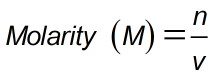Request a Tool

Molarity Calculator

Molarity (M) is the amount of a substance in a certain volume of solution

Molarity
0

Formula• n = Moles of solute
• v = Liters of solution

Defination / Uses

The amount of a material in a certain volume of solution is defined in molarity (M). The moles of a solute per litre of a method known as molarity. It is used to determine a solution's concentration.

The molarity, volume, concentration, and mass of a concentrated or dilute solution can all be calculated using a molarity calculator. Using this tool, you can obtain required responses in several units for a given word. Molarity is a term used in chemistry to describe the number of moles in one litre of solution.

The number of moles of solute must be divided by the total number of litres of solution produced to determine the molarity of a solution. If the amount of solute is given in grammes, we must first compute the number of moles of solute by using the molar mass of the solute, and then calculate the molarity by using the number of moles and total volume. Use the upper given formula for manual calculations. No sign-up, registration OR captcha is required to use this tool.